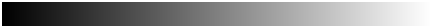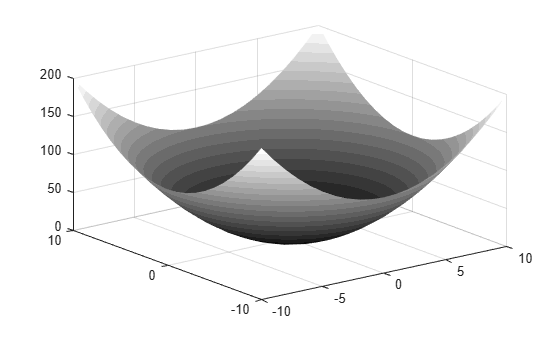# gray

Gray colormap array

•## Syntax

``c = gray``
``c = gray(m)``

## Description

example

````c = gray` returns the gray colormap as a three-column array with the same number of rows as the colormap for the current figure (`gcf`). If no figure exists, then the number of rows is equal to the default length of 256. Each row in the array contains the red, green, and blue intensities for a specific color. The intensities are in the range [0,1], and the color scheme looks like this image.```

example

````c = gray(m)` returns the colormap with `m` colors.```

## Examples

collapse all

Plot a surface and assign the gray colormap.

```surf(peaks); colormap('gray');```Get the gray colormap array and reverse the order. Then apply the modified colormap to the surface.

```c = gray; c = flipud(c); colormap(c);```Get a downsampled version of the gray colormap containing only twenty colors. Then display the contours of a paraboloid by applying the colormap and interpolated shading.

```c = gray(20); [X,Y] = meshgrid(-10:1:10); Z = X.^2 + Y.^2; surf(X,Y,Z); colormap(c); shading interp;```## Input Arguments

collapse all

Number of colors, specified as a nonnegative integer. The default value of `m` is the number of colors in the current figure's colormap. If no figure exists, the default value is `256`.

Data Types: `single` | `double`

## Version History

Introduced before R2006a

expand all